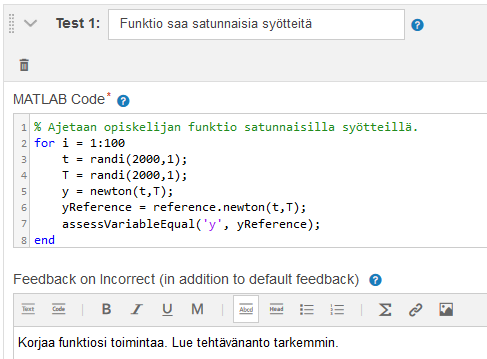This documentation explains how to create a new exercise with the proprietary MATLAB Grader service. MATLAB Grader is developed by MathWorks and it is connected to learning management systems via the standard LTI protocol, version 1.1.

One may log in directly to the MATLAB Grader at https://grader.mathworks.com/ using a MathWorks account. It is possible to host MATLAB courses there without using any LMS, but this documentation is about using it with A+. Nonetheless, teachers may create MATLAB problems in the MATLAB grader before using any LMS. When the problems are stored in a collection in the MATLAB Grader, the teacher may just copy those existing exercises when integrating the A+ LMS to the MATLAB Grader. By doing that, the teacher could first concentrate on creating the exercises and only afterwards integrate them into A+. The teacher may also use the exercises in other LTI-compatible learning management systems when they are created in MATLAB Grader collections.

## Launching a MATLAB Grader exercise from A+¶

In the A+ course, you may add new LTI exercises manually or the configurations may be imported from the MOOC grader (see previous chapters). The following describes how to add them manually under the "edit course" menu.

1. Add a new learning object

2. Choose 'LTI Exercise'

3. Enter the exercise settings and select 'MATLAB Grader' (https://lms-grader.mathworks.com/launch) as the LTI Service. LTI-specific settings "context id", "resource link id", "resource link title", and "service url" can be left empty in order to use the defaults.

4. Launch the exercise

When a teacher launches the exercise for the first time, it initializes the exercise editor in the MATLAB grader. The teacher may create a new problem or copy an existing problem from the A+ course or a MATLAB Grader collection (exercises created directly in the MATLAB Grader site without A+). In order to copy an exercise from a collection, it is necessary to link to the MathWorks account (the link for doing that should be at the bottom of the 'Add problem' page when launching the MATLAB Grader from A+). The MATLAB Grader exercise must be saved as final in order to open it to students. Exercises in the draft mode are not available to students.

The official MATLAB Grader help documentation provides more details about creating exercises, but this section gives a brief summary.

### Defining the exercise parameters in MATLAB grader¶

The MATLAB grader provides an easy-to-use user interface for teachers to develop exercises. In the MATLAB grader, there are multiple fields to define for the exercise:

• Title

• Problem Description and Instructions

• Files Referenced

• Problem Type (script or function)

• Code (Reference Solution and Learner Template)

• Assessment

More help is available by clicking ? icon next to each field name.

Next, set up required values.

In Learner template, it is possible to lock some rows (click the lock icon). It is helpful when there should be some rows that are not allowed to be edited by the student.

### Setting up the assessment¶

Assessment can be executed with four different type:

• Variable Equals Reference Solution (compares a certain variable to the same variable in the reference solution)

• Function or Keyword is Present (for example, the student's code should have an if-statement)

• Function or Keyword is Absent (for example, the student's code should not have a for-loop)

• MATLAB code (Assessment system includes three built-in functions to check if answer is correct (assessVariableEqual, assessFunctionPresence, assessFunctionAbsence)).

Examples of these methods can be found in the 'Examples' section below.

Use 'Validate Reference Solution' to check that the reference code and assessments are really working.

The image shows an example of assessment written in MATLAB code for a function type exercise. The student's function is called newton and it is called one hundred times with random input parameters. The return value is compared to the return value of the reference solution.### Examples¶

Some examples of different exercise types:

Posting submission...